Updated on 20 Oct 2021

# Sum of Squares Total, Sum of Squares Regression and Sum of Squares ErrorIliya Valchanov
Published on 5 Nov 2018 5 min read

You may be wondering what all of those sums of squares are all about. Maybe that’s what got you here in the first place. Well, they are the determinants of a good linear regression. This tutorial is based on the ANOVA framework you may have heard before.

Before reading it, though, make sure you are not mistaking regression for correlation. If you’ve got this checked, we can get straight into the action.

A quick side-note: Want to learn more about linear regression? Check out our explainer videos The Linear Regression Model. Geometrical Representation and The Simple Linear Regression Model.

## SST, SSR, SSE: Definition and Formulas

There are three terms we must define. The sum of squares total, the sum of squares regression, and the sum of squares error.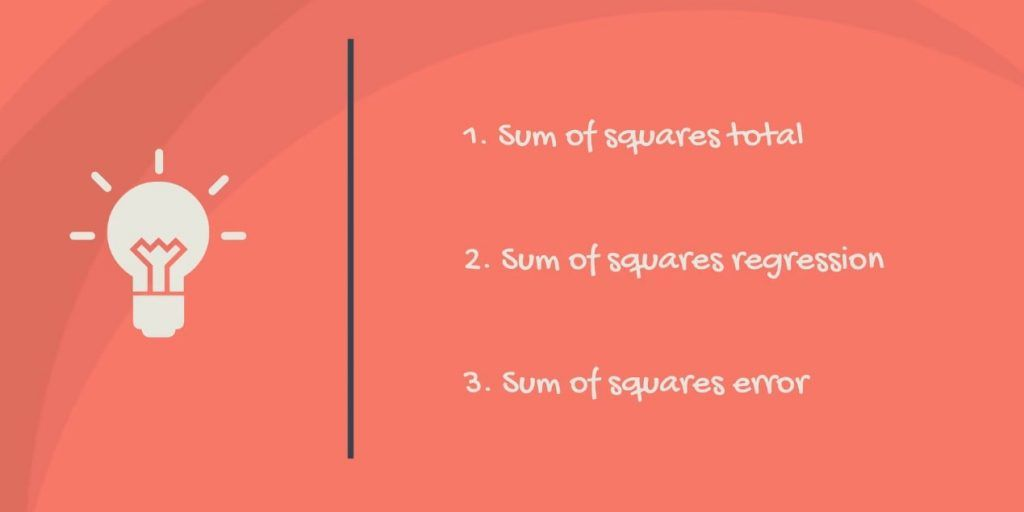### What is the SST?

The sum of squares total, denoted SST, is the squared differences between the observed dependent variable and its mean. You can think of this as the dispersion of the observed variables around the meanmuch like the variance in descriptive statistics.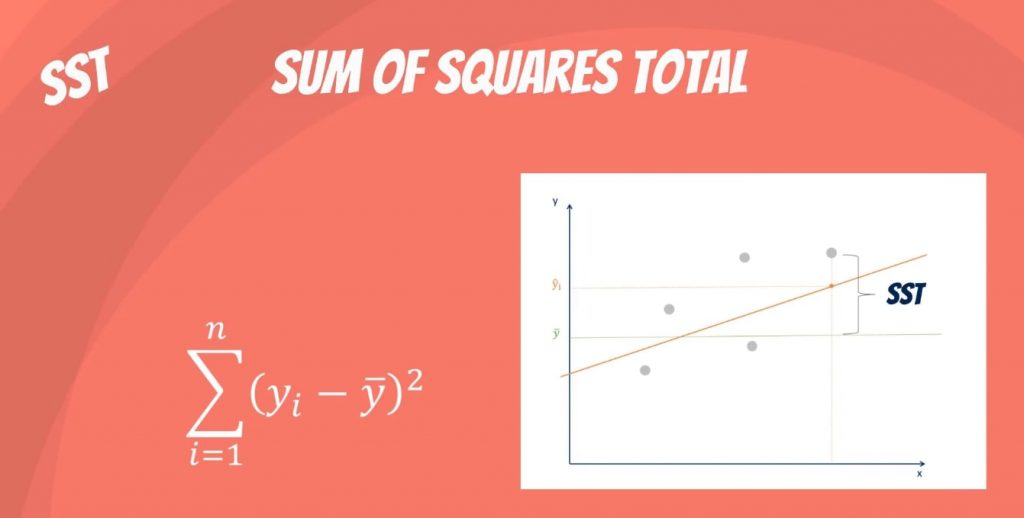It is a measure of the total variability of the dataset.

Side note: There is another notation for the SST. It is TSS or total sum of squares.

### What is the SSR?

The second term is the sum of squares due to regression, or SSR. It is the sum of the differences between the predicted value and the mean of the dependent variable. Think of it as a measure that describes how well our line fits the data.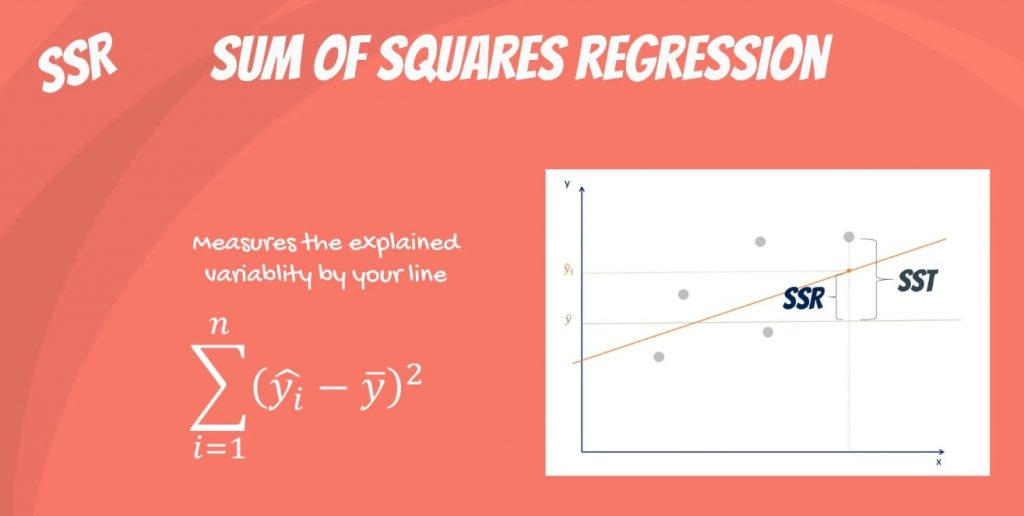If this value of SSR is equal to the sum of squares total, it means our regression model captures all the observed variability and is perfect. Once again, we have to mention that another common notation is ESS or explained sum of squares.

### What is the SSE?

The last term is the sum of squares error, or SSE. The error is the difference between the observed value and the predicted value.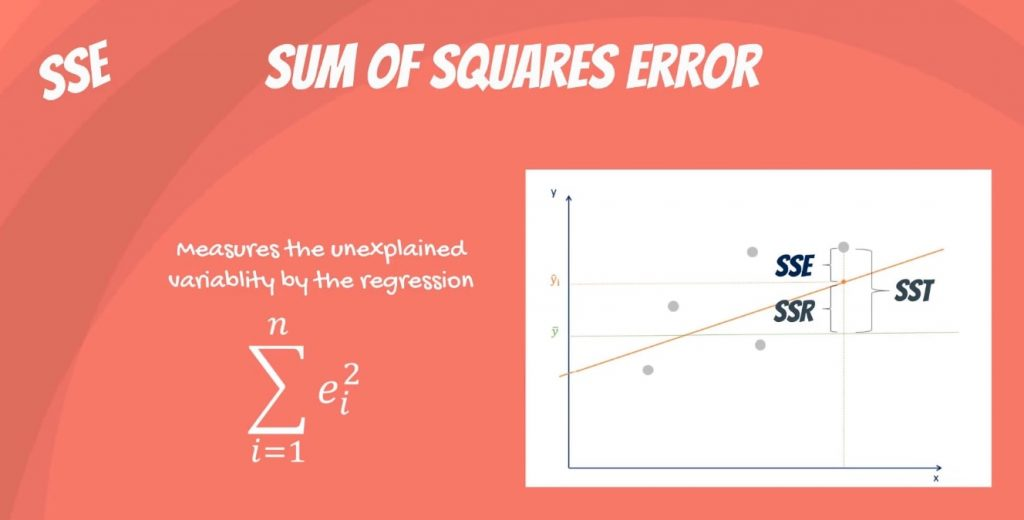We usually want to minimize the error. The smaller the error, the better the estimation power of the regression. Finally, I should add that it is also known as RSS or residual sum of squares. Residual as in: remaining or unexplained.

### The Confusion between the Different Abbreviations

It becomes really confusing because some people denote it as SSR. This makes it unclear whether we are talking about the sum of squares due to regression or sum of squared residuals.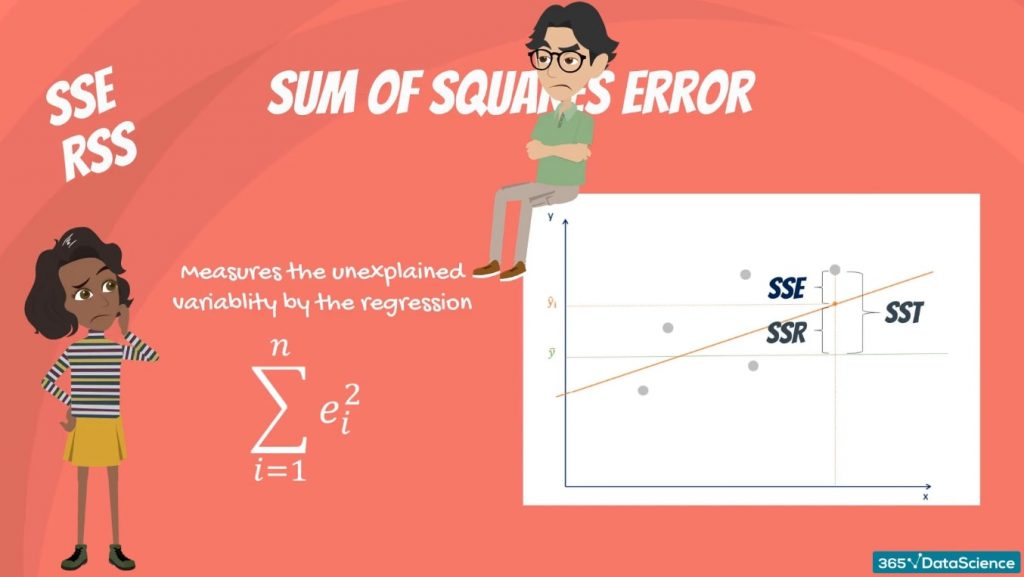In any case, neither of these are universally adopted, so the confusion remains and we’ll have to live with it.

Simply remember that the two notations are SST, SSR, SSE, or TSS, ESS, RSS.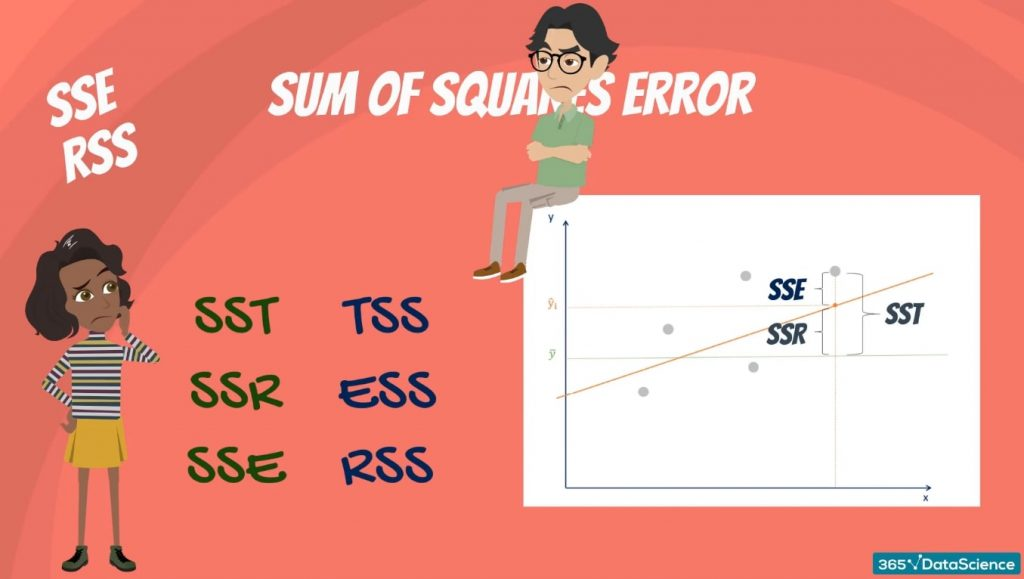There’s a conflict regarding the abbreviations, but not about the concept and its application. So, let’s focus on that.

### How Are They Related?

Mathematically, SST = SSR + SSE.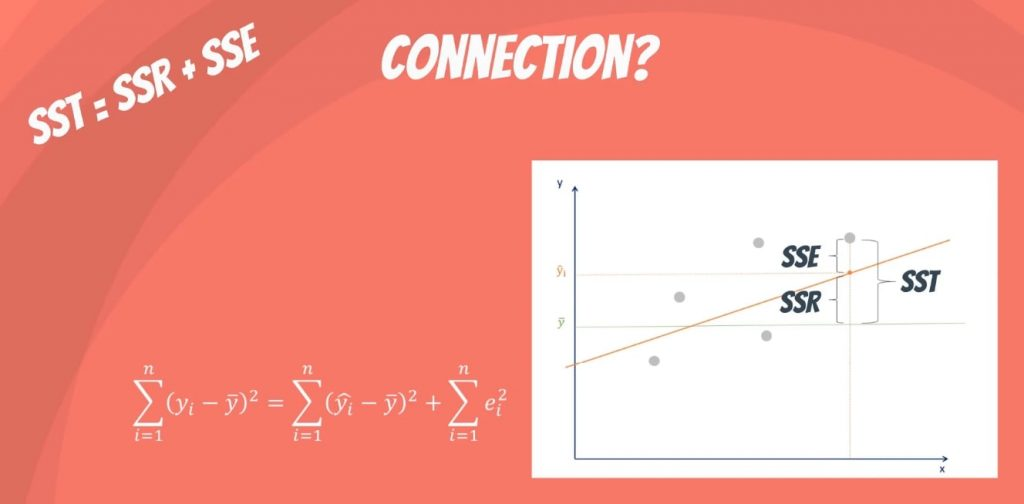The rationale is the following: the total variability of the data set is equal to the variability explained by the regression line plus the unexplained variability, known as error.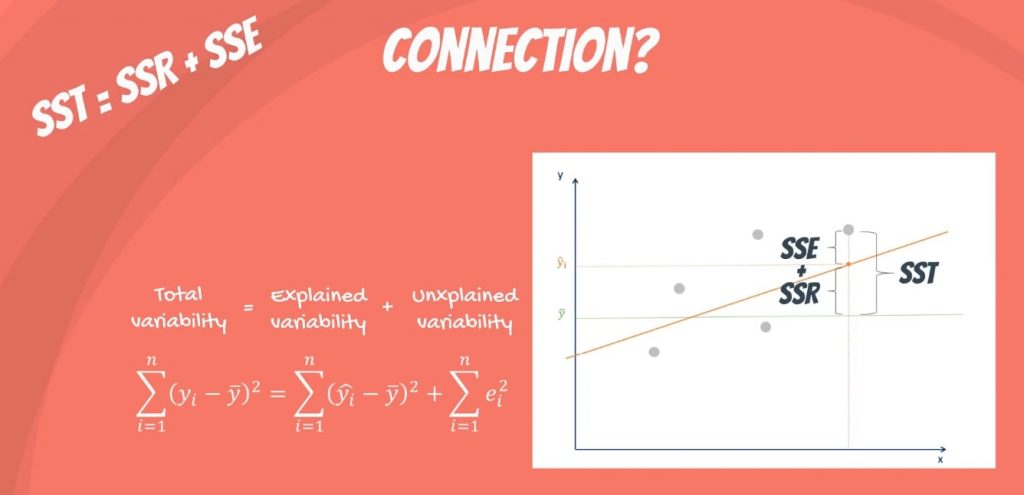Given a constant total variability, a lower error will cause a better regression. Conversely, a higher error will cause a less powerful regression. And that’s what you must remember, no matter the notation.

## Next Step: The R-squared

Well, if you are not sure why we need all those sums of squares, we have just the right tool for you. The R-squared. Care to learn more? Just dive into the linked tutorial where you will understand how it measures the explanatory power of a linear regression!

***

Interested in learning more? You can take your skills from good to great with our statistics course.

Next Tutorial: Measuring Variability with the R-squared